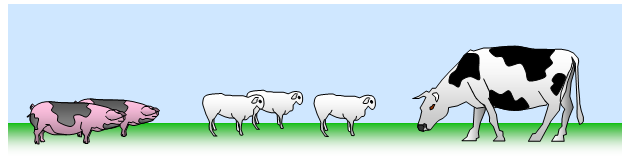# Dimensions

The basic quantities in Physics are those of mass, length, time, electric current, temperature, luminous intensity and amount of a substance. Other related quantities such as energy, acceleration and so on can be derived from combinations of these basic quantities and are therefore known as derived quantities.

The way in which the derived quantity is related to the basic quantity can be shown by the dimensions of the quantity. In considering dimensions we will restrict ourselves to those used in mechanics and properties of matter only.

The dimensions of mass are written as [M]
The dimensions of length are written as [L]
The dimensions of time are written as [T]

Note the square brackets round the letter to show that we are dealing with the dimensions of a quantity.

The dimensions of any other quantity will involve one or more of these basic dimensions. For instance, a measurement of volume of an object will involve the product of three lengths and the dimensions of volume are therefore [L]3.
In the same way a measurement of velocity requires a length divided by a time, and so the dimensions of velocity are [L][T]-1.

The table below shows the dimensions of various common quantities in mechanics.

 Quantity Dimensions area [L]2 volume [L]3 velocity [L][T]-1 acceleration [L][T]-2 force [M][L][T]-2 energy [M][L]2[T]-2 power [M][L]2[T]-3 pressure [M][L]-1[T]-2 momentum [M][L][T]-1

Dimensions have two important uses in Physics to check equations and to derive equations.

## Use of dimensions to check equations

The dimensions of the quantities of each side of an equation must match: those on the left-hand side must equal those on the right (remember the classic problem of not being able to give the total when five apples are added to three oranges, or two pigs to three sheep and one cow).For example, consider the equation: s = ut + ˝ at2

Writing this in dimensional form we have:

[L] = [L][T]- 1[T] + [L][T]-2[T]2 therefore [L] = [L] + [L]

This proves the equation, since the length on the left-hand side of the equation is obtained by adding together the two lengths on the right-hand side.

Notice that ˝ is a pure number having no dimensions and is therefore omitted in the dimensional equation.
A further example is shown below.

Example problems
Show that the equation for impulse Ft = mv - mu is dimensionally correct.
Writing this in dimensional form we have:
[M][L][T]-2[T] = [M][L][T]-1 + [M][L][T]-1
Therefore [M][L][T]-1 = [M][L][T]-1 and the equation is correct, both sides having the dimensions of momentum.

## Use of dimensions to derive equations

If we have some idea upon which quantities a further quantity might depend, then we can use the method of dimensional analysis to obtain an equation relating the relevant variables. You should appreciate that since numbers are dimensionless we cannot use this method to find these in equations, however.

Example problem
Consider the oscillation of a simple pendulum. We will assume that the period of the pendulum (t) depends in some way on the following quantities:
(i) the mass of the pendulum bob (m)
(ii) the length of the string of the pendulum (L), and
(iii) the gravitational intensity (g).

We therefore write the equation as: t = kmxLygz
where x, y and z are unknown powers and k is a dimensionless constant. Writing this in dimensional form gives:
[T] = [M]x[L]y[L]z[T]-2z
Equating the indices for M, L and T on both sides of the equation we have:
M: 0 = x L: 0 = y+z T: 1 = -2z
Therefore: x = 0, y= ˝ and z = - ˝
The original equation therefore becomes:
t = k (L/g)1/2
which is what we would expect for a simple pendulum. Dimensional analysis does not give us the value of the dimensionless constant k which can be shown by other methods to be 2p in this case.

Further examples of the use of dimensional analysis to derive equations are found in the discussions in other areas of the site
(i) viscosity - Poiseuille's equation and Stokes law
(ii) Wave velocity on a stretched string

Problems

1. Use the method of dimensional analysis to deduce equations for the following:
(a) the period of oscillation of a mass suspended on a vertical helical spring
(b) the veIocity of waves on a stretched string
(c) the frictional drag on a sphere falling through a liquid
(d) the rate at which liquid flows through a pipe

2. Use the method of dimensional analysis to check the validity of the following equations:
(a) E = mc2, where E is the energy obtainable from mass m and c is the velocity of light
(b) Energy stored in a wire = ˝EAe3/L where E is the Young modulus, A the cross-sectional area and L the original Iength
(c) Escape velocity from a planet = 2Rgo where R is the radius of the planet and go the gravitational intensity at its surface
(d) period of oscillation (T) of a floating cylinder with its axis vertical and length h immersed in a liquid of density d :
T = 2p[hd/g]˝

3. What are the dimensions for the following quantities:
(a) work
(b)energy
(c) power
(d) momentum
(e) impulse
(f) force
(g) coefficient of viscosity
(h) modulus of elasticity
(i) density
(j) coefficient of restitution

A VERSION IN WORD IS AVAILABLE ON THE SCHOOLPHYSICS CD